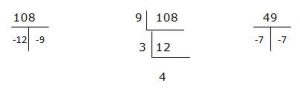## Algebra For SBI PO : Set – 09

D.1-5) In each of these questions, two equations (I) and (II) are given. You have to solve both the equation and give answer.

1)

I. (x2-10x+16)/(x2-12x+24)=2/3

II. y2-y-20=0

a) x>y

b) x<y

c) x>=y

d) x<=y

e) x=y or no relation between x and y

e)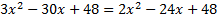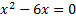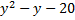y = -4, 5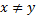2)

x2+20x +4=50-25x

y2-10y-24=0

a) x>y

b) x<y

c) x>=y

d) x<=y

e) x=y or no relation between x and y

b)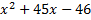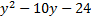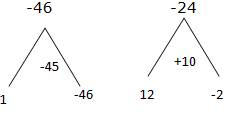3)

I. (3x-2)/y=(3x+6)/(y+16)

II. (x+2)/(y+4)=(x+5)/(y+10)

a) x>y

b) x<y

c) x>=y

d) x<=y

e) x=y or no relation between x and y

b)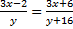3xy – 2y + 48x – 32 =3xy +6y

48x – 8y =32 —-(1)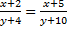xy + 2y + 10x = 20 = xy + 5y + 4x + 20

6x – 3y =0 –à (2)

48x – 8y =32

48x – 24y =0

16y =32

y =32

x = 14

x  < y

4)

I.  (x+3)(y+2)=12

II. 2xy+ 4x+ 5y = 11

a) x>y

b) x<y

c) x>=y

d) x<=y

e) x=y or no relation between x and y

e)

xy + 2x + 3y + 6 =12

2xy + 4x + 6y =12

2xy + 4x + 5y =11

_______________

y=1

6x =6

x = 1

x = y

5)

I. 8x+y=10

II. 4x+2y=13

a) x>y

b) x<y

c) x>=y

d) x<=y

e) x=y or no relation between x and y

b)

8 + y = 10

8 + 4y = 26

___________

-3y     = +6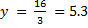42.4 + y = 10

y = 10 – 42.4

x<y

D.6-10) In each of these questions, two equations (I) and (II) are given. You have to solve both the equations and give answer.

6)

I. 3x2 – 11x + 8 = 0

II. 3y2 + 20 y + 32 = 0

a) if x>y

b) if x<y

c) if x>=y

d) if x<=y

e) if x = y or If there is no relation between ‘x’ and ‘y’.

a)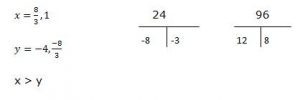7)

12x2 – 16x + 5 = 0

18y2 – 45y + 25 = 0

a) if x>y

b) if x<y

c) if x>=y

d) if x<=y

e) if x = y or If there is no relation between ‘x’ and ‘y’.

d)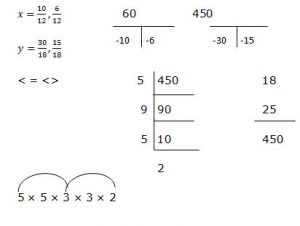8)

I. 3x2 + 5x – 8 = 0

II. y2 – 4y + 3 = 0

a) if x>y

b) if x<y

c) if x>=y

d) if x<=y

e) if x = y or If there is no relation between ‘x’ and ‘y’.

(d)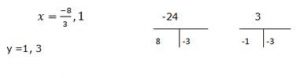9)

I. x2    – 19x + 70 = 0

II. 2y2 – 17y + 35 = 0

a) if x>y

b) if x<y

c) if x>=y

d) if x<=y

e) if x = y or If there is no relation between ‘x’ and ‘y’.

c)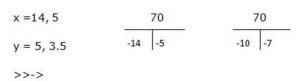10)

2x2 – 21x + 54 = 0

y2 – 14y + 49 = 0

a) if x>y

b) if x<y

c) if x>=y

d) if x<=y

e) if x = y or If there is no relation between ‘x’ and ‘y’.